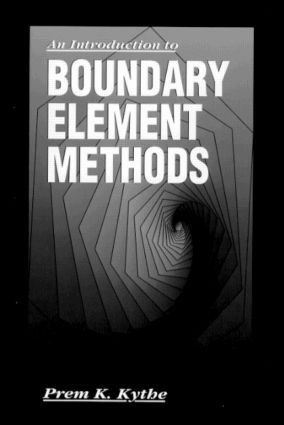An Introduction to Boundary Element Methods

1st Edition

CRC Press

384 pages

Purchasing Options:\$ = USD
Hardback: 9780849373770
pub: 1995-04-13
SAVE ~\$29.00
\$145.00
\$116.00
x

FREE Standard Shipping!

Description

The finite element and the boundary element methods are the two most important developments in numerical mathematics to occur in this century. Many engineering and mathematics graduate curricula now include a course in boundary element methods. Such a course must cover numerical methods, basic methodology to real problems, and interactive computer usage. Both theory and applications, necessary for applied courses, are available in this new textbook.

An Introduction to Boundary Element Methods is logically organized and easy to read. The topics are carefully selected and meticulously presented. Applications are described for use in identifying potential problems and for heat transfer, diffusion equations, linear elasticity, water waves, ocean acoustics, acoustic scattering, aerodynamics, porous media, and simple laminar flows.

More than 20 computer subroutines help develop and explain the computational aspect of the subject. Hundreds of figures, exercises, and solved examples supplement text and help clarify important information.

The computer programs have been tested on some benchmark problems. Even in single precision the results are more accurate and better than those obtained from available Fortran programs.

Introduction

Historical Background

What is BEM ?

Boundary Elements

Mathematical Preliminaries

Results from Calculus

Interpolation Functions

Distributions

Boundary Conditions

Dirac Delta Function

Fourier Series

Problems

References and Bibliography

Variation and Weighted Residual Methods

Weak Variational Formulation

Galerkin Method

Rayleigh-Ritz Method

Choice of Test Functions

Problems

References and Bibliography

One-Dimensional Problems

Potential Flow

Bending of an Elastic Beam

Problems

References and Bibliography

Fundamental Solutions

Eigenpairs and Dirac Delta Function

Green's Functions

Fundamental Solutions on a Finite Domain

Fundamental Solution on an Infinite Domain

References and Bibliography

Potential Problems

Laplace Equation

Boundary Elements

Constant Elements: Subroutine Be1

Linear Eelements

Discontinuous Elements: Subroutine Be2

Quadratic and Higher Elements

Poisson Equation

Non-Convex Surfaces:Subroutine Be5

Domain Integral

Unbounded Regions

Mixed Boundary Conditions

Indirect Method

Problems

References and Bibliography

Linear Elasticity

Basic Relations

Virtual Work

Somigliana Identity

Boundary Integral Equation

Derivation of BE Equation:Subroutine Bell

Torsion of a Cylindrical Bar

Three-Dimensional Medium

Axisymmetric Problems

Problems

References and Bibliography

Ocean Acoustics and Helmholtz Equation

Water Waves

Simple Amplitude Wave Theory

Helmholtz Equation

BEM:Helmholtz Equation

Underwater Acoustic Scattering

The CHIEF Algorithm

Some Structure Types

Problems

References and Bibliography

Some Fluid Flows and D'Arcy Equation

Aerodynamic Flows

Navier-Strokes Equation

Porous Media Flows

Anisotropic Porous Media

Flows with Singularities

Problems

References and Bibliography

Domain Integrals

Dual Reciprocity Method

Fourier Series Method

Multiple Reciprocity Method

Problems

References and Bibliography

Transient Problems

Transient Fourier Equation

Forward Time Marching Process

Laplace Transform BEM

Transient DRM

Transient MRM

Problems

References and Bibliography

Variational Calculus

Line Integrals

Variational Notation

Multiple Integrals

Problems

References and Bibliography

Potential Theory

Electrostatic Potential

Dirichlet Problems

Neumann Problems

References and Bibliography

One-Dimensional Logarithmic Gauss Quadrature

Two-Dimensional Gauss Quadrature Formula

References and Bibliography

Software Development

Answers to Selected Problems

Index